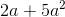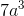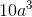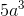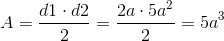## Example Questions

### Example Question #14 : How To Find The Area Of A Rhombus

A rhombus contains diagonals with the length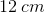and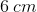. Find the area of the rhombus.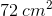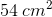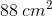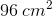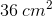Explanation:

The equation for the area of a rhombus is given by: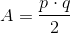whereand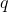are the two diagonal lengths.

This problem very quickly becomes one of the "plug and chug" type, where the given values just need to be substituted into the equation and the equation then solved. By plugging in the values given, we get: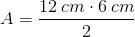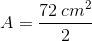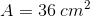### Example Question #15 : How To Find The Area Of A Rhombus

Find the area of a rhombus if the diagonals lengths are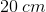and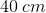.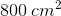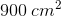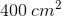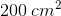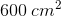Explanation:

Write the formula for the area of a rhombus: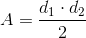Substitute the given lengths of the diagonals and solve: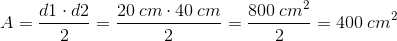### Example Question #16 : How To Find The Area Of A Rhombus

Find the area of a rhombus if the diagonals lengths are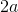and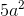.